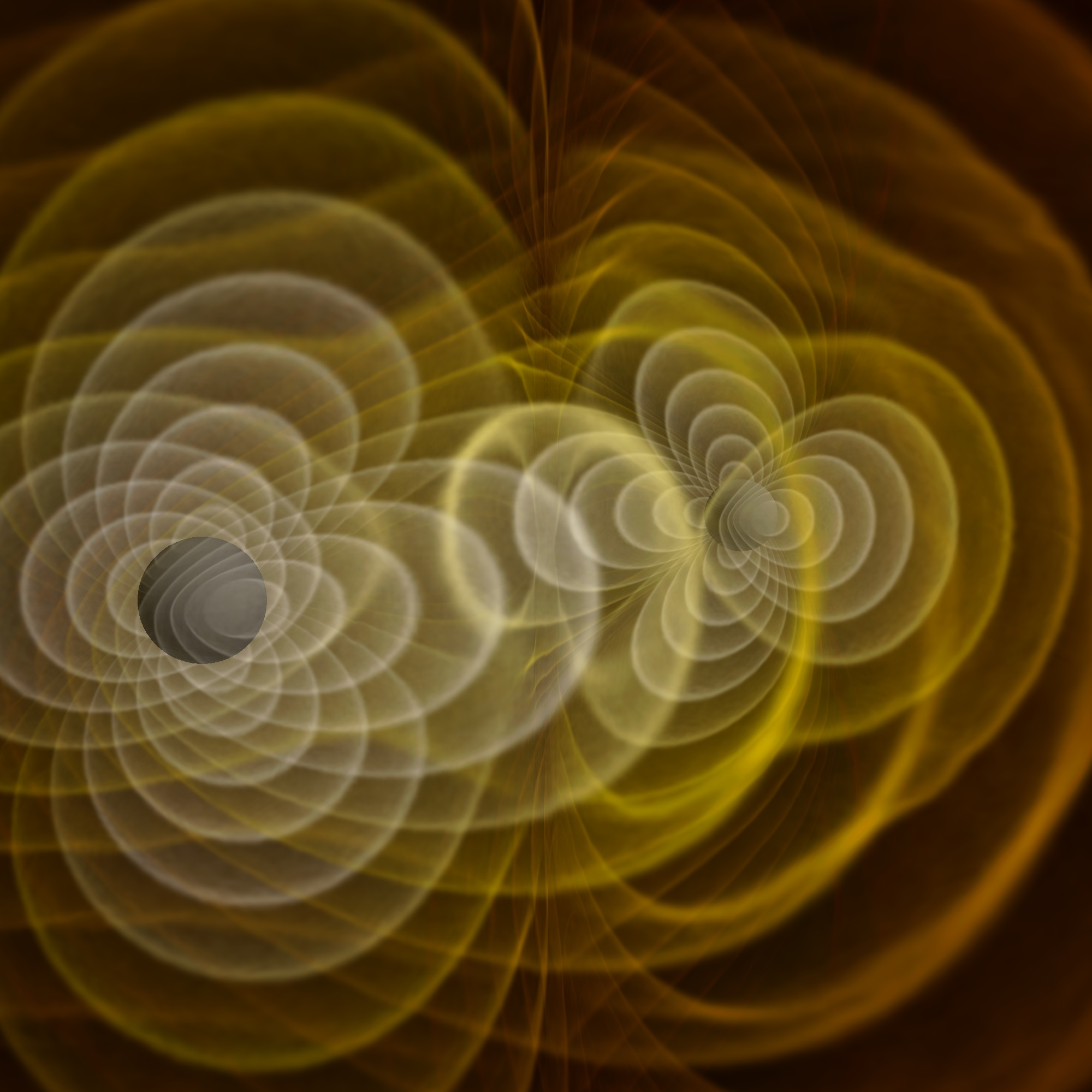# If light can't escape a black hole, how does a graviton escape?One of the most important things to keep in mind when thinking about the next Big Theory that people are developing is that physical theories are like nesting dolls. As we progress, we go to a bigger and bigger nesting doll, which is able to explain more and more things, but at its core, each theory has to explain the same set of phenomena.

So let’s take gravity - our first theory of gravity came from Newton. Newton’s laws are very basic, but they did an excellent job of explaining the way that things fall to the ground, and the way that the planets orbit the sun. These laws pretty much explain most everything we encounter in our every day lives.

However, Einstein’s theory of general relativity went a bit further, and explained that Newton’s equations could be understood as a simple way of looking at a much more complex system. Einstein’s new equations could be simplified to obtain Newton’s laws, in the small size, not very massive limit of General Relativity, but General relativity’s way of thinking about the universe provided explanations for a much wider range of objects. Many of these objects had not yet been observationally discovered, but the math Einstein put forward allows for their existence. Black holes are one of these objects. General relativity permitted objects to exist which are so dense that even light cannot escape, and many years later we found quite a bit of evidence showing that they’re real.

Gravitons come into this equation as an attempt to understand how gravity works. Gravity is kind of a mess, theoretically speaking- it just doesn’t play well with the other fundamental forces - it’s far too weak relative to the rest. We know that gravity can be explained as a curvature of space as a result of the presence of mass, but that doesn’t explain how gravity propagates. Most other forces of nature have what are called “force carriers” - little particles (or packets of energy) that obey quantum rules (in that they can pop into and out of existence) and go between two different particles as the particles interact. The force carrier particles are also called ‘virtual particles’ and, because they obey quantum rules instead of classical ones, can serve as a handy “get out of jail free” card when the classical rules seem a little restrictive. But these force carriers are predicted as part of what’s called the Standard Model.

Gravity is not part of the Standard Model. We can’t figure out how to get it in there. (We’re trying - this is how we wound up with string theory.) The quantum world and the general relativity world seem to be fundamentally incompatible. But if we’re trying to come up with a quantum-world version of gravity, then the assumption becomes that since every other force has a carrier quantum particle, gravity should have one too. And so we’ve called it the graviton, even though there’s no evidence so far that it exists, and very little information on how we think it ought to behave. If it does exist, it should at least play a role in how gravitational waves propagate.

Two more things before we can answer this question: force carriers are active at a quantum scale. This means that they will exist for extremely short periods of time, and the uncertainty principles will play a role in their behavior. Second: force carriers are only needed when there is a transfer of energy. So if a black hole were losing energy somehow (perhaps by colliding with another black hole), then the gravity waves that would be given off would involve the graviton.

But if you just had a black hole that was not losing energy, then the black hole would not be producing gravitational waves. It doesn’t change the fact that the mass of the black hole is enormous. At distances large enough to be outside of the event horizon of the black hole and scales above the quantum level, any graviton-based theory of gravity should simplify to the general relativity situation, where we can understand the mass of the black hole by the curvature of space. This curvature appears like a force because external particles respond to the local geometry of the universe. As far as gravitons go, it’s hard to understand precisely how a theoretical, highly chaotic quantum particle would behave in a region of space that has always been understood in the framework of general relativity, but however it behaves, it must result in the same solutions as general relativity.

Something here unclear, or have your own question? Feel free to ask!